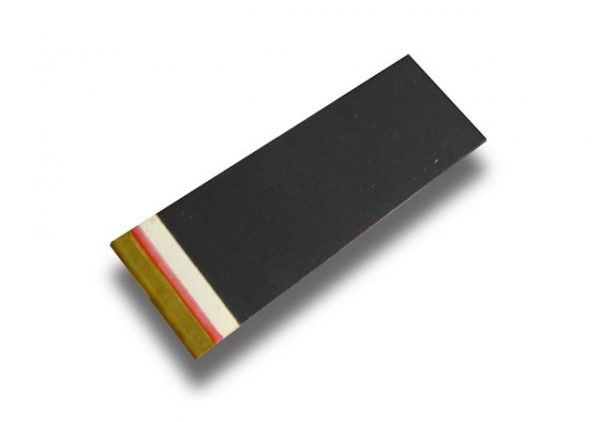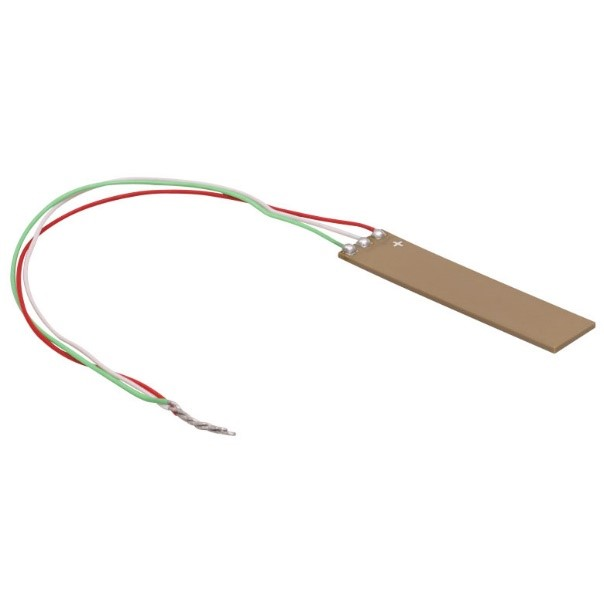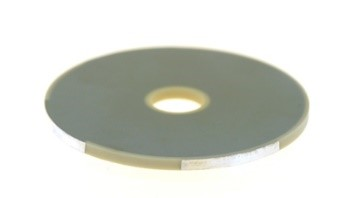# BD Family of Dual Channel Drivers for Piezo Bender Actuators

The BD family of miniature driver modules contain a high-voltage power supply and two linear amplifier channels. The individual channels can drive piezoelectric actuators up to +300V, or can be combined for a single output up to +/-300V. An inverting option allows these modules to drive two and three wire piezoelectric bender actuators.

The 3V input range, fast response, and high peak current suits applications including high speed valves, robot actuators, non-resonant piezo motors, and motion control applications.

The BD family of modules include comprehensive overload protection and are available with screw terminals or PCB mounting pins.

• #### Enquiry

Response Times are typically 2-3 days for non-technical enquiries, and 3-14 days for technical enquiries. We apologize for the delay during busy times.

• This field is for validation purposes and should be left unchanged.

• #### Obtain instant quote

• Select desired options
• Proceed to checkout
• Enter details
• Volume Pricing

### Voltage Ranges

 Variant Output Voltage Differential Output Gain Peak Current RMS Current Buy Now BD300 300 V +/- 300 V 101 50 mA 11 mA Buy Now BD250 250 V +/- 250 V 83.6 59 mA 13 mA Buy Now BD200 200 V +/- 200 V 67.7 73 mA 16 mA Buy Now BD150 150 V +/- 150 V 51.0 95 mA 21 mA Buy Now

### Operation

The modules contain a high voltage DC-DC converter and two linear amplifier channels. A range of maximum output voltages are available, from +150V to +300V. The minimum output voltage is approximately +3V, which is due to the push-pull output circuit that cannot reach exactly zero volts.

Since the minimum output is limited to approximately +3V, the minimum useful input voltage is approximately +3V/Gain. For example, the minimum useful input voltage of the BD300 is 300V/101 = 30 mV. For simplicity, this limitation is not considered in the following application examples.

Channel 2 can be switched between a non-inverting configuration (on) which is identical to channel 1, or an inverting configuration (off), which is useful when driving three wire benders or creating a single differential output.

### Application Example: Independent Channels

In this example, two 0V to 3V input signal sources produce a 0V to full-range output on two independent loads. It is recommended to connect the power supply directly to Pin 5 and 4 via twisted pair cable (if using screw terminals).

### Application Example: +/-200V Piezo Driver

This example shows a series poled bender driven in the “two-wire” configuration . A 0V to 3V input signal produces +/-200V output. Note that the load is driven differentially and cannot be connected to ground. Channel 2 is configured in the inverting mode by setting the switch to “off”. The voltage at output 1 is $V_{1}=KV_{in}$, and the voltage at output 2 is $V_{2}=K(3-V_{in})$, where $K=67.7$ for the BD200. Therefore, the voltage across the load is
$$V_{Load} = V_{1} – V_{2} = 2K(V_{in} – 1.5)$$

Therefore, a 0V input produces -200V across the load, 1.5V input produces 0V across the load, and 3V produces +200V across the load.

When the output voltage is positive, the actuator bends downward. The deflection $\delta$ is
$$\delta = 2K(V_{in} – 1.5)\frac{\delta_{pp}}{V_{pp}}$$ where $\delta_{pp}$ is the peak-to-peak displacement of the bender, and $V_{pp}$ is the maximum peak-to-peak voltage. For example, $\delta_{pp}=1.048$ mm, and $V_{pp}=360$ for the T220-A4BR-2513XB actuator www.piezo.com.

### Application Example: Three-wire Bender Driver

This example shows a parallel poled bender driven in the “biased unipolar” or “three-wire” configuration . The +150V bias voltage is achieved by configuring channel 2 in inverting mode and grounding the input.

A zero volt input results in +150V across the top piezo layer and maximum upward deflection. A +3V input results in +150V across the bottom piezo layer and maximum downward deflection. The deflection δ can be represented by
$$\delta = \frac{V_{in} – 1.5}{3}\delta_{pp}$$

where $\delta_{pp}$ is the peak-to-peak displacement of the bender. Examples of compatible actuators are listed below:

 Actuator Part Number Bias Voltage AmplifierPiezoDrive BA6020 +150 V BD150Thorlabs PB4NB2W +150 V BD150Noliac CMBR02 (see note 1) +200 V BD200

Note 1: The recommended +100V and -100V bias voltages can be replaced by +200V and 0V

### Other Specifications

 Specification Value Notes Input voltage range 0 V to 3 V Minimum useful input voltage is 3V/Gain. Input impedance 10 $k\Omega$, 5 $k\Omega$ Channel 1 is 10 $k\Omega$, Channel 2 is 5 $k\Omega$ Minimum output voltage < 3 V Output voltage does not reach zero Over-current protection Peak and RMS Includes direct short circuit Thermal protection 70 C Disabled when PCB temp > 70 C Power supply +12 V to +30 V +24 V recommended Supply power 5 W Maximum average power under full load Small signal bandwidth 20 kHz Slew rate 12 V/us Output noise < 1% of full range E.g. < 3 Vpp for BD300 Status pin voltage 0.6 V < 0.5 V signals a thermal overload Temperature range 0 C to +50 C Humidity Non-condensing Dimensions 60 X 25.4 mm 2.3 X 1 inch Height 8 mm Total height required for PCB mount version Weight 11 g

### Output Current

The peak and average current limit specifications are listed in “Voltage Ranges”. The RMS current limit defines the maximum frequency that is achievable with a capacitive load, which is discussed in “Power Bandwidth”.

The peak current can be drawn for up to five milliseconds before the RMS current limit engages. This allows fast step changes with capacitive loads. The output voltage slew rate is:
$$\frac{\Delta V_{o}}{\Delta t} = \frac{i_{pk}}{C_{L}}$$ Where $i_{pk}$ is the peak current limit, $C_{L}$ is the load capacitance, $\Delta V_o$ is the change in output voltage, and $\Delta t$ is the time interval. For example, a voltage change of $\Delta V$ will require a slewing time of $\Delta t = \Delta V_{o} C_{L}/i_{pk}$.

### Power bandwidth

The power bandwidth is the maximum frequency periodic signal that can be reproduced without distortion. The online power bandwidth calculator determines the maximum operating frequency and required power for a given load capacitance. The calculator includes the effects of current limit, slew-rate, and signal bandwidth.

The calculator does not include the time-constant of the peak current limit, and therefore may become inaccurate when the power bandwidth is below 50 Hz.

### Signal Bandwidth and Settling Time

With a load capacitance below 10 nF, the small signal bandwidth of the BD modules is 20 kHz. The rise-time with a step input is approximately 20 us.

With a load capacitance above 10 nF, the bandwidth and rise-time are effected by the output impedance, current limit, and voltage slew-rate. For periodic signals, refer to the online calculator above. For step-response rise times, refer to “Output Current”.

### Heat Dissipation

When driving capacitive loads, the heat dissipation of the module is equal to the required supply power calculated by the online calculator. The worst case is approximately 5W which may require some enclosure ventilation for passive convection. The PCB temperature can be monitored by measuring the voltage on the test point labelled “Temp”. The temperature in degrees C is
$$T = \frac{V_{temp} – 0.55}{1.96 \times 10^{-3}} + 25$$

### Status Pin

In normal operation, the status pin voltage is 0.6V. If the voltage drops below 0.5V, this indicates that the PCB temperature is above 70C and the power supply has been temporarily disabled.

The status pin can also be pulled low to disable the high-voltage power supply. For example, by using the circuit below:

### PCB Footprint

An Altium schematic and footprint library is available for download. The recommended schematic symbol and footprint are:

### High Voltage Safety Warning

This product produces potentially lethal voltages up to 330 Vdc.

Observe Low-Voltage (as per ANSI C84.1-1989) safety precautions, e.g.

• Use an observer trained in low-voltage rescue
• Do not operate with exposed conductors
• Use appropriate signage

### References

 A New Electrical Configuration for Improving the Range of Piezoelectric Bimorph Benders; S. A. Rios, A. J. Fleming; Sensors and Actuators A: Physical, 2015.